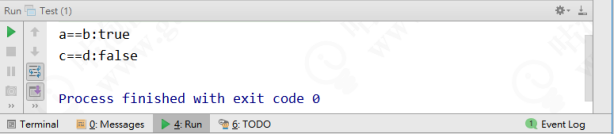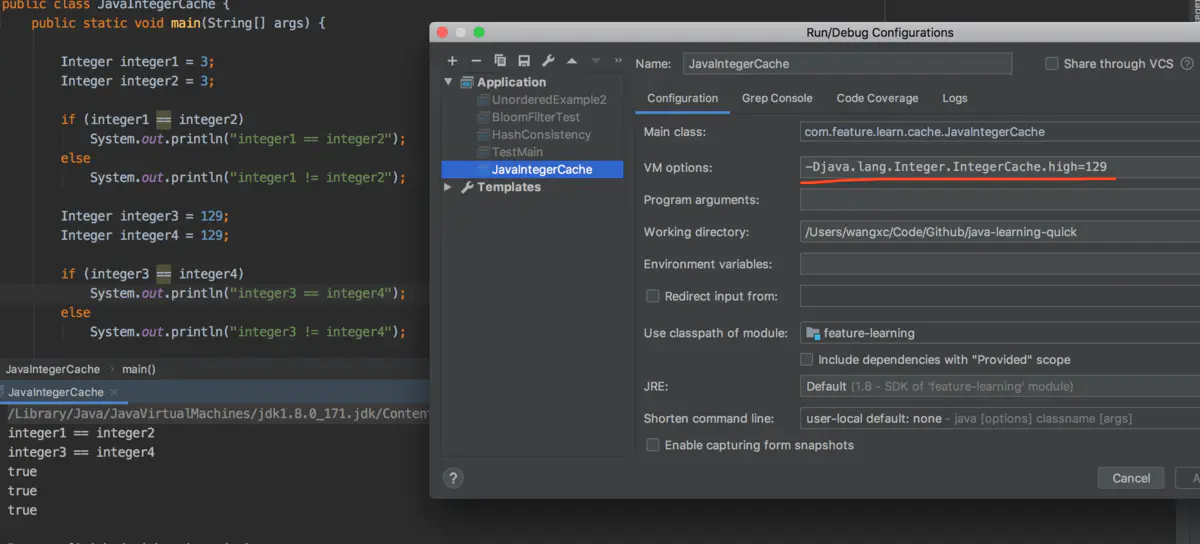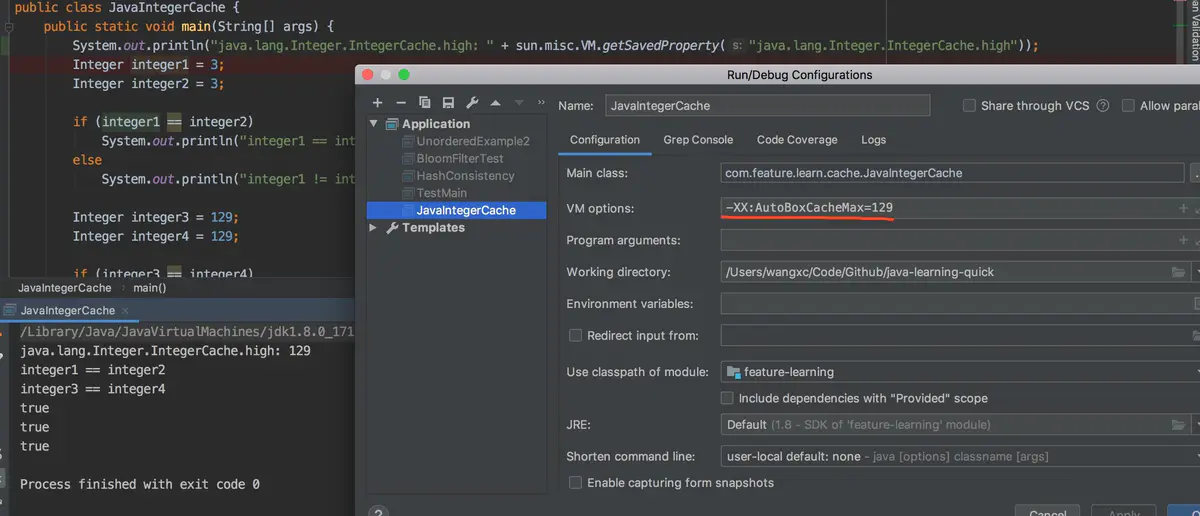# 就因为把 int 改成 Integer，第 2 天被辞了Tom弹架构

## 1 故事背景

public static void main(String[] args) {        Integer a = Integer.valueOf(100);        Integer b = 100;        Integer c = Integer.valueOf(129);        Integer d = 129;        System.out.println("a==b:" + (a==b));        System.out.println("c==d:" + (c==d));}public final class Integer extends Number implements Comparable<Integer> {    ...    public static Integer valueOf(int i) {            if (i >= IntegerCache.low && i <= IntegerCache.high)                return IntegerCache.cache[i + (-IntegerCache.low)];            return new Integer(i);    }    ...}

private static class IntegerCache {  // 最小值  static final int low = -128;  // 最大值，支持自定义  static final int high;  // 缓存数组  static final Integer cache[];  static {    // 最大值可以通过属性配置来改变    int h = 127;    String integerCacheHighPropValue =      sun.misc.VM.getSavedProperty("java.lang.Integer.IntegerCache.high");    // 如果设置了对应的属性，则使用该值    if (integerCacheHighPropValue != null) {      try {        int i = parseInt(integerCacheHighPropValue);        i = Math.max(i, 127);        // 最大数组大小为Integer.MAX_VALUE        h = Math.min(i, Integer.MAX_VALUE - (-low) -1);      } catch( NumberFormatException nfe) {        // If the property cannot be parsed into an int, ignore it.      }    }    high = h;            cache = new Integer[(high - low) + 1];    int j = low;    // 将low-high范围内的值全部实例化并存入数组中当缓存使用    for(int k = 0; k < cache.length; k++)      cache[k] = new Integer(j++);    // range [-128, 127] must be interned (JLS7 5.1.7)    assert IntegerCache.high >= 127;  }  private IntegerCache() {}}

Returns an Integer instance representing the specified int value. If a new Integer instance is not required, this method should generally be used in preference to the constructor Integer(int), as this method is likely to yield significantly better space and time performance by caching frequently requested values. This method will always cache values in the range -128 to 127, inclusive, and may cache other values outside of this range

128～127 的数据在 int 范围内是使用最频繁的，为了减少频繁创建对象带来的内存消耗，这里其实是用到了享元模式，以提高空间和时间性能。

JDK 增加了这一默认的范围并不是不可变，我们在使用前可以通过设置-Djava.lang.Integer.IntegerCache.high=xxx 或者设置-XX:AutoBoxCacheMax=xxx 来修改缓存范围，如下图：Java Language Specification 的原文解释如下：

Ideally, boxing a given primitive value p, would always yield an identical reference. In practice, this may not be feasible using existing implementation techniques. The rules above are a pragmatic compromise. The final clause above requires that certain common values always be boxed into indistinguishable objects. The implementation may cache these, lazily or eagerly. For other values, this formulation disallows any assumptions about the identity of the boxed values on the programmer's part. This would allow (but not require) sharing of some or all of these references.This ensures that in most common cases, the behavior will be the desired one, without imposing an undue performance penalty, especially on small devices. Less memory-limited implementations might, for example, cache all char and short values, as well as int and long values in the range of -32K to +32K.

## 2 关于 Integer 和 int 的比较

1. 由于 Integer 变量实际上是对一个 Integer 对象的引用，所以两个通过 new 生成的 Integer 变量永远是不相等的（因为 new 生成的是两个对象，其内存地址不同）。

Integer i = new Integer(100);Integer j = new Integer(100);System.out.print(i == j); //false

1. Integer 变量和 int 变量比较时，只要两个变量的值是向等的，则结果为 true（因为包装类 Integer 和基本数据类型 int 比较时，java 会自动拆包装为 int，然后进行比较，实际上就变为两个 int 变量的比较）

Integer i = new Integer(100);int j = 100；System.out.print(i == j); //true

1. 非 new 生成的 Integer 变量和 new Integer()生成的变量比较时，结果为 false。（因为 ①当变量值在-128 - 127 之间时，非 new 生成的 Integer 变量指向的是 java 常量池中的对象，而 new Integer()生成的变量指向堆中新建的对象，两者在内存中的地址不同；②当变量值在-128 - 127 之间时，非 new 生成 Integer 变量时，java API 中最终会按照 new Integer(i)进行处理（参考下面第 4 条），最终两个 Interger 的地址同样是不相同的）

Integer i = new Integer(100);Integer j = 100;System.out.print(i == j); //false

1. 对于两个非 new 生成的 Integer 对象，进行比较时，如果两个变量的值在区间-128 到 127 之间，则比较结果为 true，如果两个变量的值不在此区间，则比较结果为 false

## 4 使用享元模式实现数据库连接池

public class ConnectionPool {    private Vector<Connection> pool;    private String url = "jdbc:mysql://localhost:3306/test";    private String username = "root";    private String password = "root";    private String driverClassName = "com.mysql.jdbc.Driver";    private int poolSize = 100;public ConnectionPool() {        pool = new Vector<Connection>(poolSize);        try{            Class.forName(driverClassName);            for (int i = 0; i < poolSize; i++) {                Connection conn = DriverManager.getConnection(url,username,password);                pool.add(conn);            }        }catch (Exception e){            e.printStackTrace();        }    }    public synchronized Connection getConnection(){        if(pool.size() > 0){            Connection conn = pool.get(0);            pool.remove(conn);            return conn;        }        return null;    }    public synchronized void release(Connection conn){        pool.add(conn);}}## 评论